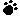Please review your test results by moving cursor to answer sheet icon.1.A)
B)
C)
D)

Your answer=, Correct answer=,

2.An estimating technique that uses a statistical relationship between historical data and other variables (for example, square footage in construction, lines of code in software development) is known as:

A) Parametric Estimating
B) Analogous Estimating
C) Bottom-up Estimating
D) Historical AnalysisYour answer=B, Correct answer=, Work Sheet:

3.Your project is mid-way through a delivery schedule. As project manager, you want to understand how much work is left. Which is the most accurate way to determine the remaining work to be done by the project team?

A) Rolling wave method
B) Earned Value Technique
C) A Manual forecast
D) Future analysisYour answer=A, Correct answer=, Work Sheet:

4.A)
B)
C)
D)

Your answer=, Correct answer=,

5.A project is estimated to cost \$ 50,000 with a timeline of 50 days. After 25 days, the project manager finds that 50% of the project is complete and Actual costs are \$ 50,000. What is the Cost Performance Index (CPI) ?

A) The CPI is 1
B) The CPI is 1.5
C) The CPI is 2
D) The CPI is 0.5Your answer=C, Correct answer=, Work Sheet: